Next:7.6 To Probe FurtherUp:7.5 Directed Acyclic GraphsPrevious:7.5.2 Topological Sort

## 7.5.3 Strong Components

REF.
Rao S. Kosaraju. Unpublished. 1978.
REF.
Robert E. Tarjan. Depth first search and linear graph algorithms. SIAM Journal on Computing, Volume 1, Number 2, pp. 146-160, 1972.
• A strongly connected component of a digraph is a maximal set of vertices in which there is a path from any one vertex to any other vertex in the set. For an example, see Figure 7.15.

•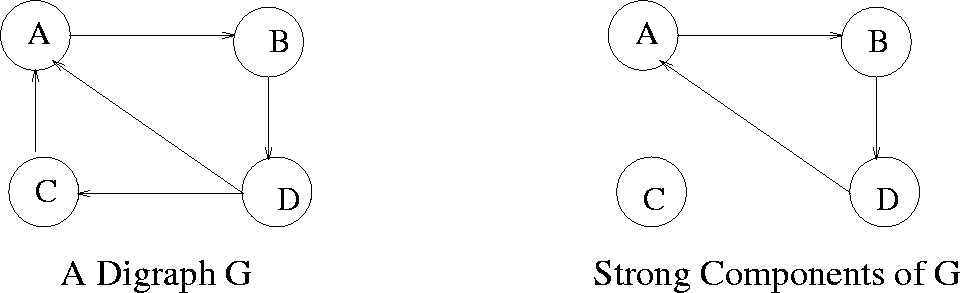• Let G = (V, E) be a digraph. We can partition V into equivalence classes Vi, 1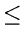ir, such that vertices v and w are equivalent if there is a path from v to w and a path from w to v.

• Let Ei, 1ir, be the set of arcs with head and tail both in Vi.

Then the graphs (Vi, Ei) are called the strong components of G.

A digraph with only one strong component is said to be strongly connected.

• Depth-first-search can be used to efficiently determine the strong components of a digraph.
• Kosaraju's (1978) algorithm for finding strong components in a graph:
• 1.
Perform a DFS of G and number the vertices in order of completion of the recursive calls.
2.
Construct a new directed graph Gr by reversing the direction of every arc in G.
3.
Perform a DFS on Gr starting the search from the highest numbered vertex according to the numbering assigned at step 1. If the DFS does not reach all vertices, start the next DFS from the highest numbered remaining vertex.
4.
Each tree in the resulting spanning forest is a strong component of G.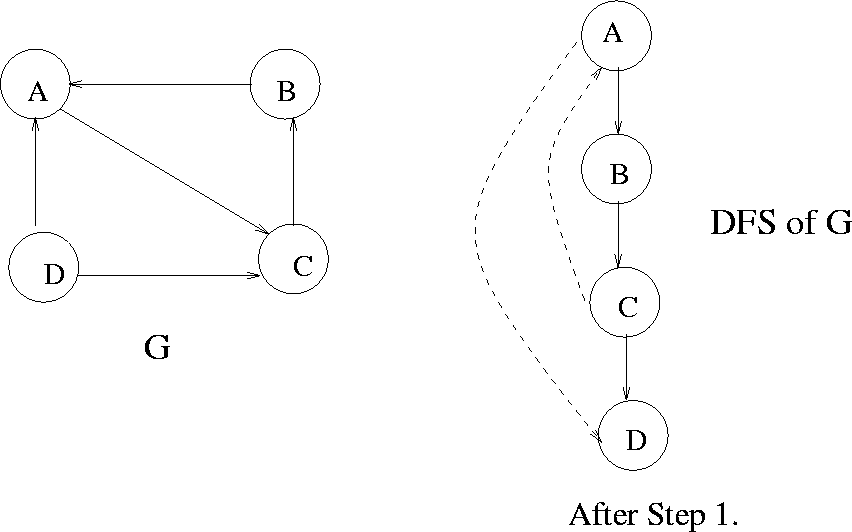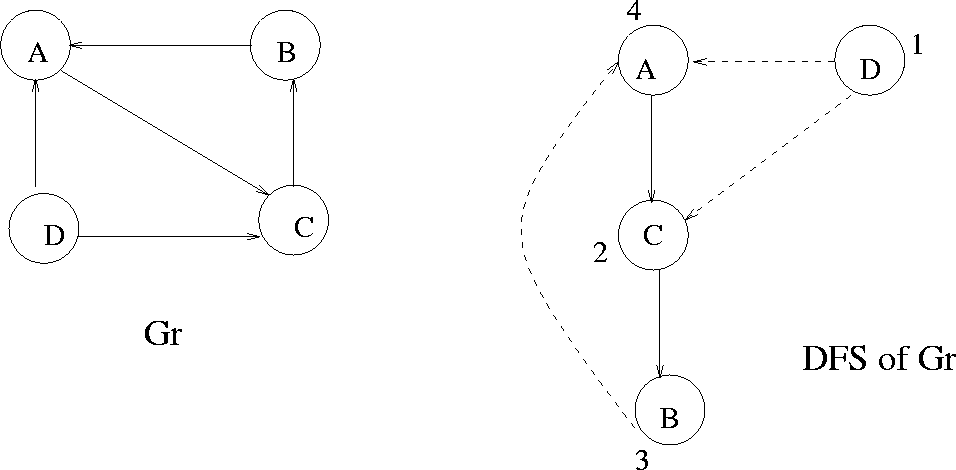Example: See Figures 7.16 and 7.17

Proof of Kosaraju's Algorithm

• First we show: If v and w are vertices in the same strong component, then they belong to the same spanning tree in the DFS of Gr.

• v and w in the same strong component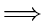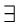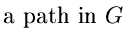from v to w and from w to vfrom w to v and from v to w
Let us say in the DFS of Gr we start at some x and reach vor w. Since there is a path from v to w and vice versa, v and wwill end up in the same spanning tree (having root x).
• Now we show: If v and w are in the same spanning tree in the DFS of Gr, then, they are in the same strong component of G.

• Let x be the root of the spanning tree containing v and w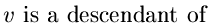x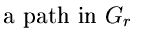from x to vfrom v to x
In the DFS of Gr, vertex v was still unvisited when the DFSat x was initiated (since x is the root)x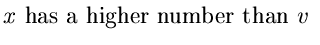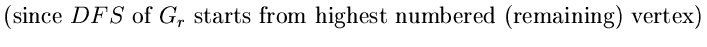.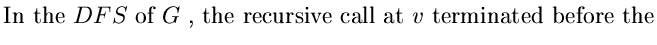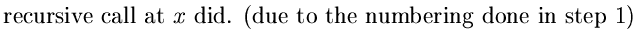u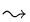x (7.16) +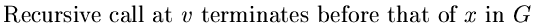(7.17)

In the DFS of G, there are two possibilities.

 1)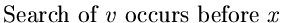(7.18) 2)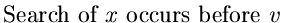(7.19)

 (1),(3)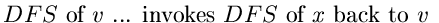.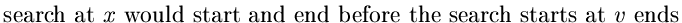.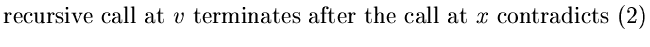.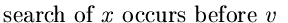. (4),(2)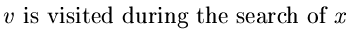.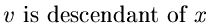.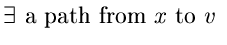.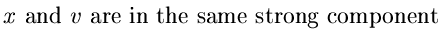. Similarly,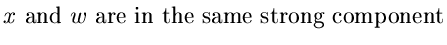.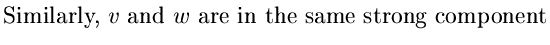.Next:7.6 To Probe FurtherUp:7.5 Directed Acyclic GraphsPrevious:7.5.2 Topological Sort
eEL,CSA_Dept,IISc,Bangalore